# The Duoprisms

Mathematically speaking, the duoprisms are simply Cartesian products of two polygons. Specifically, an m,n-duoprism is the Cartesian product of an m-sided polygon with an n-sided polygon. The resulting duoprism consists of all the points whose X and Y coordinates are in the m-sided polygon and whose Z and W coordinates are in the n-sided polygon.

This definition, while precise, is rather difficult to visualize, so we shall employ a different, more geometrically-motivated line of description.

### Construction

Consider the following cell-first perspective projection of the 4D hypercube: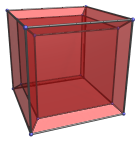If we take the outer cube, the inner cube, and the upper and lower frustums, we have a cycle of cubical cells in the hypercube.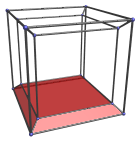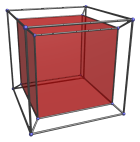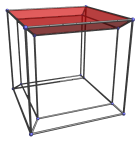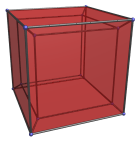This cycle lies in the ZW plane.

Notice how the remaining four cells, the left, right, front, and back frustums, form another cycle, in the XY plane. These two cycles are mutually perpendicular.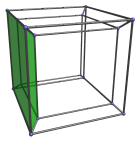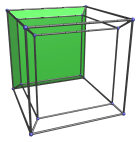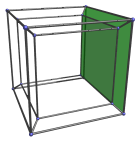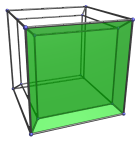Now, examine the first cycle in the ZW plane. Each member of this cycle is connected to the two adjacent members by their top and bottom square faces.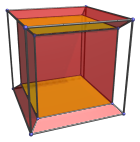The 4 remaining faces of each member are not connected to another member of this cycle, but to members of the XY cycle. Furthermore, each of these remaining faces corresponds precisely with one member of the XY cycle.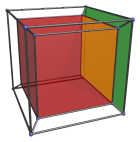This suggests that we can substitute the cubes in the ZW cycle with, for example, pentagonal prisms. The pentagonal faces are attached to each other within the cycle, leaving the 5 surrounding square faces free. This means that we can add a 5th cube to the XY cycle, and we get a closed object consisting of 4 pentagonal prisms and 5 cubes. This we call a 5,4-duoprism.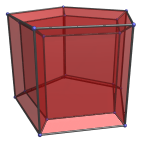This is not all. Notice that the reason the XY cycle consists of cubes is because the square faces of the ZW cycle form loops of 4 squares each. If we add more pentagonal prisms to the ZW cycle, they will form a larger loop of squares: in other words, they will trace out a larger prism in the XY cycle. For example, we can make the ZW cycle have 6 pentagonal prisms; this then dictates that the XY cycle must be made of 5 hexagonal prisms. This we call a 5,6-duoprism.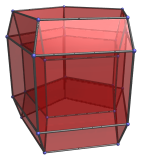This is a general procedure that works with XY and ZW cycles of any size greater than 2. In general, if we use n-gonal prisms in the ZW cycle, then there must be n members in the XY cycle. Analogously, if there are m members in the ZW cycle, then the XY cycle must consist of m-gonal prisms. We call the resulting shape an m,n-duoprism.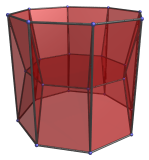The above is an 8,3-duoprism. Note that an m,n-duoprism is identical to an n,m-duoprism. They are simply rotated versions of each other.

### Specific Duoprisms

More detailed information is available for the following specific duoprisms:

Last updated 05 Apr 2019.